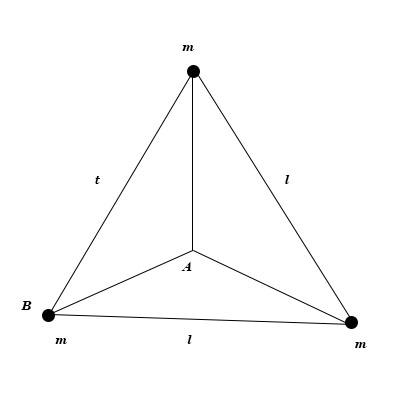# Three equal masses m are rigidly connected to each other by massless rods of length l forming and...

## Question:

Three equal masses {eq}m {/eq} are rigidly connected to each other by massless rods of length {eq}l {/eq} forming and equilateral triangle, as shown below. The assembly is to be given an angular velocity {eq}\omega {/eq} about an axis perpendicular to the triangle. For fixed {eq}\omega {/eq}, what is the ratio of the kinetic energy of the assembly for an axis through {eq}B_t {/eq} compared with that for an axis through {eq}A. {/eq}## Rotational Kinetic Energy:

The kinetic energy of rotational motion can be determined in the way similar for the way to determine translational kinetic energy. The rotational kinetic energy equals the one half of the product of rotational inertia and angular velocity squared.

The kinetic energy of rotating system is given by:

{eq}K = \dfrac {I\omega^2}{2} {/eq}

Here

• {eq}I {/eq} is the rotational inertia of the system;

For the rotation about point B, we have:

{eq}I_B = 2 m l^2 {/eq}

The rotational inertia about the point A. we have:

{eq}I_A = 3 m AB^2 {/eq}

For the length of AB, we obtain the following equation:

{eq}l^2 = 2 AB^2 - 2 AB^2 cos (120^{\circ}) = 3 AB^2 {/eq}

Therefore. we obtain:

{eq}I_A = ml^2 {/eq}

Thus, the ratio of kinetic energies is:

{eq}\dfrac {K_B}{K_A} = \dfrac {I_B}{I_A} = \dfrac {2ml^2}{ml^2} = 2 {/eq}